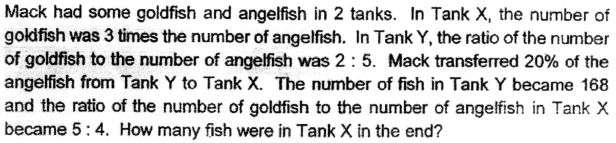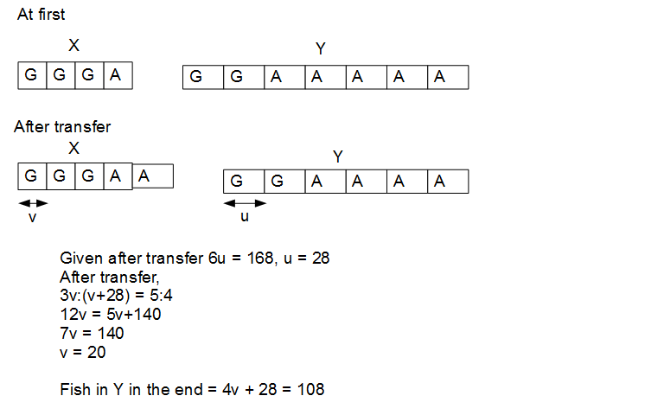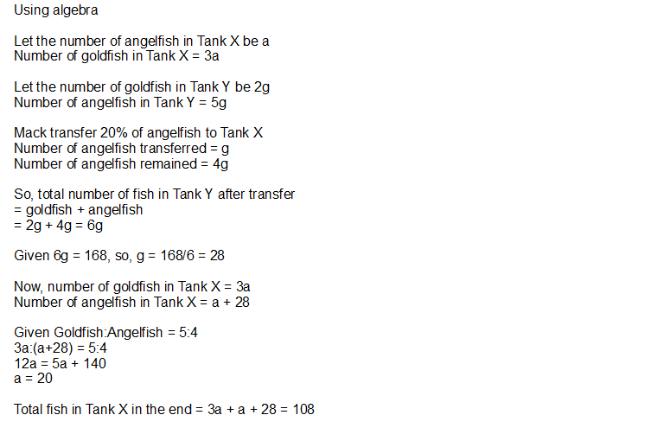# Questionpls kindly explain in algebra method. thank you so much.

5 Answers

# Answer

 Tank X Tank Y (-trfr) End in X Rework units in Tank X G 3u = 15q 2p 5r = 15q Know that no. of Goldfish in X remains the same. Let it be 15q (LCM of 3 and 5) A 1u   +1p =5q + 28 5p     -1p 4r =12q 12q=  5q + 28 Total 168  = 6p p=28 27q See above and deduce 7q=28 q=4 Total in Tank X 27q = 108

1 Reply 0 Likes ✔Accepted Answer

thank you so much

0 Replies 0 Likes

In Tank X,

goldfish : angelfish
3u : 1u

In Tank Y,
goldfish : angelfish
2p : 5p

Mack transferred 20% of the angelfish from Tank Y to tank X.
100% – 20% = 80%
2p + 80% x 5p = 6p ——- 168
1p —— 168/6 = 28

3u/(1u + 28) = 5/4
(1u + 28) x 5 = 5u + 140 ——- 3u x 4 = 12u
1u ——- 140/(12 – 5) = 20
4u + 28 ——- 4 x 20 + 28 = 108

Ans : 108 fish.

0 Replies 0 Likes

This is Before After question.

In Tank X,

LCM of 3 and 5 is 15, goldfish no change

3 : 1 = 15 : 3

5 : 4 = 15 : 12

 Tank X Goldfish Angelfish total Before 15u 5u After 15u 12u 27u

20% = 12u – 5u = 7u

100% = 7u × 5 = 35u

 Tank Y Goldfish Angelfish Before 14u 35u After 14u 28u

14u + 28u = 168

42u = 168

1u = 4

27u = 27 × 4 = 108

0 Replies 0 Likes

This is Before After question.

In Tank X,

LCM of 3 and 5 is 15, goldfish no change

3 : 1 = 15 : 3

5 : 4 = 15 : 12

 Tank X Goldfish Angelfish total Before 15u 5u After 15u 12u 27u

20% = 12u – 5u = 7u

100% = 7u × 5 = 35u

 Tank Y Goldfish Angelfish Before 14u 35u After 14u 28u

14u + 28u = 168

42u = 168

1u = 4

27u = 27 × 4 = 108

0 Replies 0 Likes1 Reply 0 Likes

pls kindly explain in algebra method. as i’m not familiar with model drawing method. thankyou.

1 Reply 0 Likes1 Reply 0 Likes

thanks for your detail explanation.

0 Replies 0 Likes